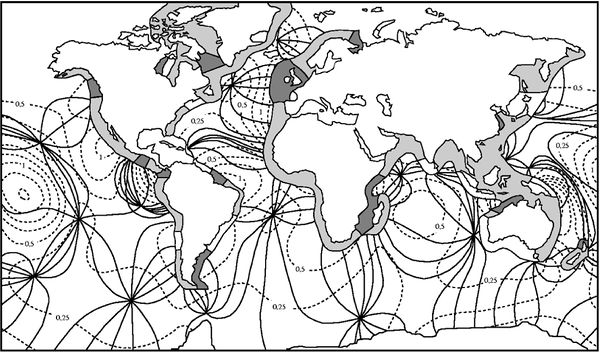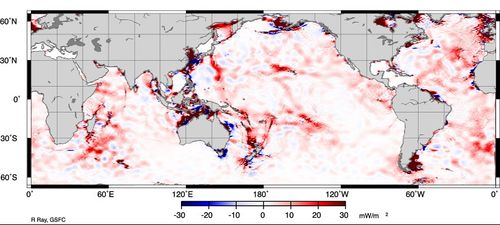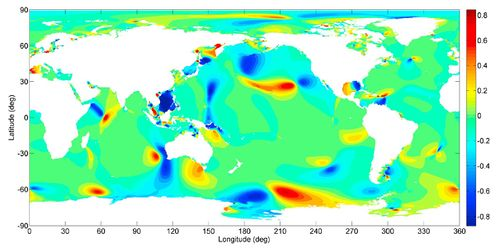# Difference between revisions of "Ocean and shelf tides"

In this article the term tide refers to the astronomical tide.

## Introduction

Tidal motion is the oscillation of ocean waters under the influence of the attractive gravitational forces of the moon and the sun. The response of the ocean to the gravitational forces follows a pattern of rotating ('amphidromic') systems, as a consequence of earth's rotation. The frequencies are determined by the relative periodic motions of moon, sun and earth surface. The amplitude of the tidal oscillation is very small compared to ocean depths. The ocean tidal oscillation in each point can therefore be represented by a linear superposition of sinusoidal tidal components with frequencies corresponding to the different cycles of the gravitational forces. The most important tidal components have a periodicity which is close to semidiurnal or diurnal, due to earth's rotation.

## Tide generation

### Moon and sun

The regular daily upward and downward motion of the water surface along the coastline is the most visible expression of tidal forcing. In ancient Greece it was recognized that tides are in some way related to sun and moon, but this relationship could not be explained . The explanation of tidal motion as resulting from gravitational forces was given by Newton. The lunar and solar tide generating forces acting on the oceans are extremely small; they are about a factor $\, 10^{-7} \,$ smaller than the gravitational force of the earth. The tide-generating force of the moon is about twice as large as that of the sun.

### Tidal wave and ocean basin resonance

Ocean tides owe their strength for a large part to resonance; tidal motion is amplified for tidal components with a frequency close to the frequency of free oscillations in the ocean basins. This is the case in particular for the semi-diurnal tide in the North Atlantic and Pacific Oceans , and also for the diurnal tide in the Pacific Ocean . Tidal waves in wide ocean basins (width typically larger than a few thousand km) rotate around points of zero amplitude, the amphidromic points; the rotation is counterclockwise in the Northern Hemisphere and clockwise in the Southern Hemisphere. This due to earth's rotation, which induces Coriolis acceleration of tidal currents. Fig.1 shows the semi-diurnal tidal wave in the world's oceans; it comprises a large number of amphidromic systems, where the tide rotates in resonance with the semi-diurnal component of the gravitational force. This ocean tidal wave pattern can be described in first approximation by a combination of Kelvin and Sverdrup waves (also called Poincaré waves, see Tidal motion in shelf seas).Fig. 1. System of semidiurnal lunar tidal waves ($M_2$) in the oceans represented by lines of equal tidal phase (solid, intervals of 30$^{\circ}$) and lines of equal tidal amplitude (dashed, intervals of 0.25 m). Three ranges are indicated for the tidal amplitude $a$ on the continental shelf: microtidal (white fringe, $a \lt$ 1 m), mesotidal (light grey fringe, 1 $\lt a \lt$ 2 m) and macrotidal (dark grey fringe, $a \gt$ 2 m). Redrawn after Bearman (1991) 

### Tidal Energy Dissipation

The total power available from Moon and Sun on the global ocean is estimated at 3.7 TW ($10^{12}$ Watts); after allowing for a comparatively small dissipation in the atmosphere and the solid Earth, 3.5 TW remain to be dissipated in the ocean (Munk and Wunsch, 1998). For comparison, the geothermal heat loss is 30 TW, and the equator-to-pole ocean heat-flow is 2,000 TW. Solar radiation input (175,000 TW) is five orders of magnitude greater than the tidal power.Fig. 2. Estimates of $M_2$ tidal energy dissipation based on six years of altimeter data from the TOPEX/Poseidon satellite. The blue patches (negative dissipation) are clearly not physical, and provide some indication of the magnitude of noise in the estimates (Egbert and Ray, 2000). Image from NASA.

The tidal energy dissipation due to the $M_2$ tide can be exactly calculated from astronomic observation programs at 2.5 TW, of which 0.1 TW is dissipated in the atmosphere and the solid Earth (Munk, 1997). It is estimated that 1.8 TW of $M_2$ energy (2.5 TW of all lunar and solar constituents) is lost in the turbulent bottom boundary layers of marginal seas due to the work against bottom friction which opposes tidal currents. This kind of energy dissipation is concentrated in a few shelf areas of strong tidal current, such as the north-west European Shelf, the Patagonian Shelf, the Yellow Sea, the Timor and Arafura Seas, and the Amazon Shelf (Fig. 2). The other 0.6 TW of $M_2$ tidal dissipation (1.0 TW of all lunar and solar constituents) occurs in the open ocean through the scattering by ocean-bottom topography of surface tides into internal tidal waves (Egbert and Ray, 2000). The breaking of internal waves may be concentrated in a few areas, generally near rugged bottom topography, for example seamounts and mid-ocean ridges (Fig. 2), and is believed to be a principal contributor to drive the ocean interior mixing (Munk and Wunsch, 1998).

### Modelling ocean tides

Modelling ocean tides remains a challenge, even with modern fast computers. Because the tidal wavelength is much larger than ocean depth, these models are generally based on the hydrostatic shallow-water equations. Relatively simple models considering the response of a homogeneous ocean to the tide-generating forces yield results in qualitative agreement with observations. However, for better quantitative agreement stratification and internal tide generation (at ocean ridges, in particular) should be included. Other factors that need to be taken into account are:

• Tide-induced seafloor deformation under the weight of the water column (loading tide);
• Tide-induced seafloor deformation related to the solid earth tide;
• Gravitational attraction induced by the mass of the ocean on the ocean itself (self-attraction).

See Hendershott (2007)  for a discussion of these different factors.

## Tidal components

### Semidiurnal periodicity

In order to explain tidal motion, it is not sufficient to consider only the gravitational forces exerted by moon and sun on the earth's water masses. Tide generation results from the local imbalance at the earth's surface of two opposing factors: the gravitational forces acting between the earth and the moon (and between the earth and the sun), and the centrifugal acceleration related to the orbital motions of these celestial bodies. In fact, only the tangential component of the gravitational force is relevant - water is not accelerated away from the earth's surface. Because the gravitational force and the centrifugal acceleration are in balance at any location on the earth's surface twice during each diurnal rotation, the major periodicity is approximately semidiurnal (a little more than 12 hours because of moon's orbital motion).

### Diurnal tide and spring-neap cycle

Because the solar and lunar orbits do not coincide with the equatorial plane (the angle between orbital plane and equatorial plane is called declination), a daily inequality arises in the semidiurnal cycle. The daily inequality is strongest in shelf seas that resonate at diurnal frequency and which are situated close to amphodromic points of the semidiurnal tide. In such regions the tide is mainly diurnal.

Due to the $\approx$ 30-day orbital motion of the moon, the moon-earth and sun-earth axes approximately coincide every 15 days (syzygy). This causes a 15-day cycle of neap tide and spring tide; spring tide follows full moon and new moon and neap tide follows half-moon (with a delay of one or two days due to frictional dissipation ). In fact, the 15-day period corresponds to the frequency difference of the semidiurnal lunar component ($M_2$) and the semidiurnal solar component ($S_2$); the interference of these components produces the neap-spring variation of the tidal amplitude.

### Other tidal components

The different cycles in the relative motions of moon, sun and earth surface generate tidal waves with corresponding periods. The lunar semidiurnal tidal component ($M_2$, period $\approx$ 12 h 25 min) is generally the largest tidal constituent (see Fig. 3), followed by the semidiurnal solar tide $S_2$ (period = 12 h). Other important tidal constituents are:

• the diurnal component $K_1$, which is related to the declination of the lunar and solar orbits relative to the equatorial plane (period $\approx$ 24 h),
• the diurnal lunar component $O_1$ (period $\approx$ 26 h) and
• the diurnal solar component $P_1$ (period $\approx$ 24 h).

There are many other tidal components of smaller magnitude and lower frequency related to periodicity in the lunar and terrestrial orbits. A modulation of the mean tidal amplitude of the order of 5% is related to the 18.6 year oscillation in the declination of the lunar orbit.

### Prediction of tides

Because the astronomical tide responds to a cyclic forcing by the gravitational motions of earth, moon and sun (including earth's rotation), tides are a cyclic phenomenon at any place on earth and can be predicted by analysing observations from the past. Therefore the amplitude and phase of all relevant tidal components need to be determined. The most usual method today is based on the least-squares technique . The tidal elevation is represented by a sum of the (sinusoidal) tidal components that are expected to yield a significant contribution. The amplitudes and phases of the tidal components are free parameters which are determined by a least-square fit of the representation to the observed tidal elevation record. The tidal record should be sufficiently long to eliminate meteorological influences. The observation record should also be longer (at least a few times) than the largest period appearing in the representation. For resolving two components with close periods $T$ and $T+\Delta T$ the length of the observation record should exceed a few times $\; T^2 / \Delta T$. For many stations around the world tidal predictions are available (see for example https://maree.shom.fr/) .

### Asymmetric ocean tides

Because of the minor role of friction in the propagation of ocean tides, individual astronomic tidal components are well described by sinusoidal functions. This implies that for each component the rising and falling branches are symmetric. This also holds on average for a superposition of tidal components, if the frequencies $\omega_i$ of the major tidal components are not linearly related with integer coefficients ($\omega_i$ is different from any combination of sums and differences of $\omega_j, \; j \neq i$). Taken over a sufficiently long time the average period of rising tide then equals the average period of falling tide. Hence, at the continental shelf boundary there is symmetry between the astronomical forcing of flood currents and ebb currents.Fig. 4. World map of tidal asymmetry. The colors represent a measure of the difference in duration between falling tide and rising tide. A positive value close to 1 (red) represents a much steeper tidal rise than tidal fall and a large negative value close to -1 (blue) represents a much steeper tidal fall than tidal rise. The precise definition of the tidal asymmetry indicator is given in the original publication by Song et al. (2011).

However, certain combinations of astronomic components yield asymmetric tides, irrespective of the averaging period. The reason is that most tidal constituents result from a superposition of a limited number of basic cycles in the relative motions of earth, moon and sun. The frequencies of these tidal constituents correspond to sums and differences of the basic frequencies . This implies that different tidal constituents may interfere in such a way that flood and ebb are modulated in a systematic, asymmetric way. Such asymmetries may become significant in the case of strong diurnal tides ; for dominant semidiurnal tides this effect is small. Fig. 4 shows a world map of tidal asymmetry computed according to the tidal constants derived from TPXO7-ATLAS by Song et al. (2011).

A particular example is the combination of the $M_2, K_1$ and $O_1$ tides. The sum of the frequencies of the $K_1$ and $O_1$ tidal constituents equals the frequency of the $M_2$ tidal constituent. In regions where the amplitudes of the $K_1$ and $O_1$ tides are not small compared to the amplitude of the $M_2$ tide, the sum of these three tidal constituents yields an asymmetric tide, with an asymmetry depending on the relative phase of these tidal constituents. Along the Californian coast, for example, the combination of $M_2, K_1$ and $O_1$ yields a tidal asymmetry with longer duration of rising tide compared to falling tide . Duration asymmetry of tidal rise and tidal fall induces an asymmetry in the strength of flood and ebb currents in tidal inlets. This has consequences for residual sediment transport and for the morphology of the inward tidal basins (see Tidal asymmetry and tidal basin morphodynamics and Morphology of estuaries for more details).

## Tides on the continental shelf and in coastal basins

### Tidal amplification

The tides generated in the ocean propagate to the continental shelf, where the tidal range may increase further. Different phenomena contribute to this amplification: resonant dimensions of the shelf sea, slowing down of wave-energy propagation ('shoaling') or concentration of the tidal energy flux in areas of reduced width ('funneling'). The maximum spring tidal range can exceed 14 m in some funnel-shaped bays, such as the Bay of Fundy (Canada), Bristol Channel (UK) and Baie du Mont Saint Michel (France).

Tidal amplification in shelf seas is counteracted by frictional momentum dissipation. The strong tidal motion occurring in many shelf seas is thus not locally produced by tide generating forces, but results from co-oscillation with ocean tides and from local topographic amplification. Numerical tidal studies for the northwest European shelf  and for the East China shelf  show that the local tide generating force influences the co-oscillating semidiurnal tide in these shelf seas by no more than about 1%. Tidal resonance in shelf seas generally has a damping effect on ocean tides .

### Higher harmonic components

Tides on the continental shelf are due to co-oscillation with the ocean tides at the continental shelf boundaries, as noted earlier. However, the non-linearity of tidal propagation in shallow environments (water depth no much greater than ten times the tidal amplitude, or less) alters the sinusoidal character of the ocean tide at the shelf boundary. The distortion of the tidal wave can be represented by additional tidal components corresponding to multiples (overtides) or superposition (compound tides) of the dominant ocean tidal frequencies . An important consequence of tidal wave distortion is the asymmetric character of tidal motion in shallow water: the periods of tidal rise and tidal fall are not equal. This asymmetry is represented in particular by a quarter-diurnal tidal component (indicated by M4), which induces different strengths of flood currents and ebb currents. In Fig. 5, coastal regions are indicated with an important M4 tidal component; they coincide largely with coastal zones where the semidiurnal tide is strong (Fig. 3).

## Tides in estuaries and tidal lagoons

The tidal wave is further distorted when entering coastal inlets and estuaries. This is due the shallowness of these systems (the water depth is in many cases no greater than a few times the tidal amplitude or even less) and due to interaction with the basin topography (for example, expansion of the tidal flood wave over intertidal flats). As a consequence of tidal asymmetry, substantial differences may arise between the strength of flood and ebb currents. This results in a net import or export of sediment and corresponding sedimentation or erosion. The basin morphology will evolve and tidal asymmetry will change consequently. This morphodynamic feedback process is described in more detail in the article Tidal asymmetry and tidal basin morphodynamics. Tidal distortion in some estuaries and shallow tidal lagoons may become so strong that the tidal flood wave evolves into a tidal bore. This process is described in more detail in the article Tidal bore dynamics.

## Related articles

Tidal motion in shelf seas
Coriolis acceleration
Tidal asymmetry and tidal basin morphodynamics
Morphology of estuaries
Tidal bore dynamics